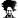# In a set of 3, find the average of 2 lowest numbers and average of 2 highest numbers then average the results

#### johnstandy

##### New Member
 186 62 44
When you have 3 numbers such as this, how can you find the average of the two lowest numbers (62, 44) and then the average of the two highest numbers (186, 62) so that you can then take the average of those two averages.

62+44=106 106/2=53
186+62=124 248/2=124

53+124=177 177/2=88.5

I have a huge set of data and I'm looking for a formula that will accomplish this if anyone has any ideas

Thanks

#### John Jairo V

##### Well-Known Member
Hi, @johnstandy !

If you have your numbers in A1:C1, you could try:

=AVERAGE(AVERAGE(LARGE(A1:C1,{1,2})),AVERAGE(LARGE(A1:C1,{2,3})))
or
=AVERAGE(MMULT(LARGE(A1:C1,{1,2;2,3}),{1;1})/2)

Blessings!

•Thomas Kuriakose

#### johnstandy

##### New Member
Hi, @johnstandy !

If you have your numbers in A1:C1, you could try:

=AVERAGE(AVERAGE(LARGE(A1:C1,{1,2})),AVERAGE(LARGE(A1:C1,{2,3})))
or
=AVERAGE(MMULT(LARGE(A1:C1,{1,2;2,3}),{1;1})/2)

Blessings!
It worked. Thank you so much!

#### XOR LX

##### Active Member
Hi,

Another:

=MMULT(LARGE(A1:C1,{1,2,3}),1/{4;2;4})

Regards

#### Peter Bartholomew

##### Well-Known Member
A bit of preliminary math:
= SUM(triple)/4 + MEDIAN(triple)/4

#### Attachments

• 14.9 KB Views: 9
•Thomas Kuriakose and XOR LX

#### XOR LX

##### Active Member
Very nice! @Peter Bartholomew
Not sure how I missed your extra step. From [(a1+a2)/2+(a2+a3)/2]/2 (where we can assume that a1<=a2<=a3 without loss of generality), I arrived at (a1+2a2+a3)/4, but failed to see that this was equivalent to (a1+a2+a3)/4 + a2/4.
Regards

Last edited:

#### p45cal

##### Well-Known Member
In Peter's file:
=AVERAGE(B4:D4,MEDIAN(B4:D4))
or
=AVERAGE(triple,MEDIAN(triple))

#### Peter Bartholomew

##### Well-Known Member
@XOR LX
I like array formulae (I once suggested that it is the array formula that should be committed with Enter leaving those who want to break the array down to suffer the pain of Ctrl+Enter) but MMULT is not the nation's favourite and explaining it can be heavy going. Hence the extra math step that you noted.

I also checked out a SUMPRODUCT version of your formula
=SUMPRODUCT({1;2;1}, LARGE(triple, {1;2;3}) / 4

#### Peter Bartholomew

##### Well-Known Member
This is of no relevance to the OP but I found myself wondering what the best approach might be to the problem of averaging top and bottom pairs of an arbitrary sized set of numbers. There are many possibilities but one I liked was to define a Named formula 'extremes' to refer to
= IF( {0,1}, LARGE(numbers, {1;2}), SMALL(numbers, {1;2}) )
The result is then given by
= AVERAGE(extremes)

Since we appear to have a group of contributors that are comfortable with array formulas, here are a few thoughts on array formulas that have made an impression on me, together with my recollection of from whom I have heard an authoritative statement.

All calculation within Excel is performed by an array calculation engine - Joe McDaid
There is no separate calculation code that operates with scalars, they are simply 1x1 arrays.

References to arrays within the cell are reduced to single values by implicit intersection - Joe McDaid
CSE is no more that an instruction to omit this additional step.

All Names are references to array formulas - Daniel Ferry
They may be thought of as named ranges or named constants but they are still just formulas.

#### XOR LX

##### Active Member
Interesting generalisation. Another option (Excel 2010 and later):

=AVERAGE(AGGREGATE({14,15},,numbers,{1;2}))

Regards

#### Peter Bartholomew

##### Well-Known Member
=AVERAGE(AGGREGATE({14,15},,numbers,{1;2}))
I like it#### GraH - Guido

##### Well-Known Member
=AVERAGE(AGGREGATE({14,15},,numbers,{1;2}))
Needed some time to understand this. Truly